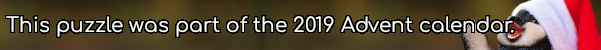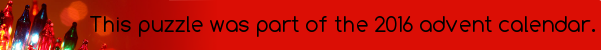mscroggs.co.uk
mscroggs.co.uksubscribe

# Puzzles

## 24 DecemberThere are six 3-digit numbers with the property that the sum of their digits is equal to the product of their digits. Today's number is the largest of these numbers.

## 6 DecemberNoel's grandchildren were in born in November in consecutive years. Each year for Christmas, Noel gives each of his grandchildren their age in pounds.
Last year, Noel gave his grandchildren a total of £208. How much will he give them in total this year?

## 20 DecemberWhat is the largest number that cannot be written in the form $$10a+27b$$, where $$a$$ and $$b$$ are nonnegative integers (ie $$a$$ and $$b$$ can be 0, 1, 2, 3, ...)?

## Square pairs

Source: Maths Jam
Can you order the integers 1 to 16 so that every pair of adjacent numbers adds to a square number?
For which other numbers $$n$$ is it possible to order the integers 1 to $$n$$ in such a way?

## 14 DecemberIn July, I posted the Combining Multiples puzzle.
Today's number is the largest number that cannot be written in the form $$27a+17b$$, where $$a$$ and $$b$$ are positive integers (or 0).

## Combining multiples

In each of these questions, positive integers should be taken to include 0.
1. What is the largest number that cannot be written in the form $$3a+5b$$, where $$a$$ and $$b$$ are positive integers?
2. What is the largest number that cannot be written in the form $$3a+7b$$, where $$a$$ and $$b$$ are positive integers?
3. What is the largest number that cannot be written in the form $$10a+11b$$, where $$a$$ and $$b$$ are positive integers?
4. Given $$n$$ and $$m$$, what is the largest number that cannot be written in the form $$na+mb$$, where $$a$$ and $$b$$ are positive integers?

## Subsum

1) In a set of three integers, will there always be two integers whose sum is even?
2) How many integers must there be in a set so that there will always be three integers in the set whose sum is a multiple of 3?
3) How many integers must there be in a set so that there will always be four integers in the set whose sum is even?
4) How many integers must there be in a set so that there will always be three integers in the set whose sum is even?

## Santa

Each of the letters D, A, Y, S, N, T, B, R and E represents a different non-zero digit. The following sum is true:
$$\begin{array}{cccccc} D&A&D&D&Y\\ B&E&A&R&D&+\\ \hline S&A&N&T&A \end{array}$$
This has a unique solution, but I haven't found a way to find the solution without brute force. This less insightful sum is also true with the same values of the letters (and should allow you to find the values of the letters using logic alone):
$$\begin{array}{ccccc} R&A&T&S\\ N&E&R&D&+\\ \hline S&A&N&E \end{array}$$

## Archive

Show me a random puzzle
▼ show ▼